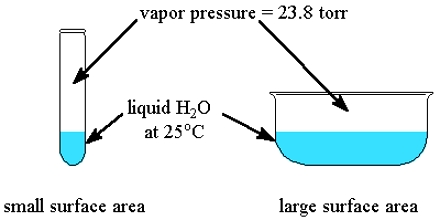Chemistry

# Vapor pressure of liquid### Vapor pressure of liquid:

At definite temperature the pressure exerted by vapor above the liquid, which is in equilibrium with the liquid is called the vapor pressure of the liquid. With increase of temperature vapor pressure of substance also increases and at definite temperature when the vapor pressure of liquid becomes equal to the atmospheric pressure above it then liquid substance start to boil with bubbles.

The vapor pressure of liquid substance on the basis of  kinetic theory of gas:

We know at definite temperature the pressure exerted by vapor above the liquid, which is in equilibrium with the liquid is called the vapor pressure of the liquid. From the kinetic theory of gas we know every matter has two kinds of energy viz. Potential energy and kinetic energy. When this two types of energy are almost equal then the matter become a liquid. This liquid turns into gas when the kinetic energy becomes greater than potential energy.

Kinetic energy of a substance at liquid means the average value of energy due to the movement of molecules of matter. In liquid state though kinetic energy of most the molecules is equal to the average energy but in every liquid kinetic energy of few molecules is less as more than average kinetic energy. Those molecules whose individual kinetic energy is greater than average kinetic energy and potential energy they sometimes come in the upon of matter and go out by overcoming the potential energy and become vapor. This process of liquid substance is called vaporization. If heat is applied to liquid substance kinetic energy of molecules increase, i.e. Kinetic energy of more molecules is greater than potential energy; therefore rate of conversion into vapor also increases.

From this it can be understood that process of vaporization depends on temperature. Rate of vaporization increase with the increase of temperature and rate decreases if temperature decreases. With the increase of temperature vapor pressure of a substance also increases and at a definite temperature when vapor pressure of liquid become equal to the atmospheric pressure above it then liquid substance start to boil with bubbles. The temperature at which vapor pressure of liquid becomes equal to the atmospheric pressure is called boiling point of the liquid.